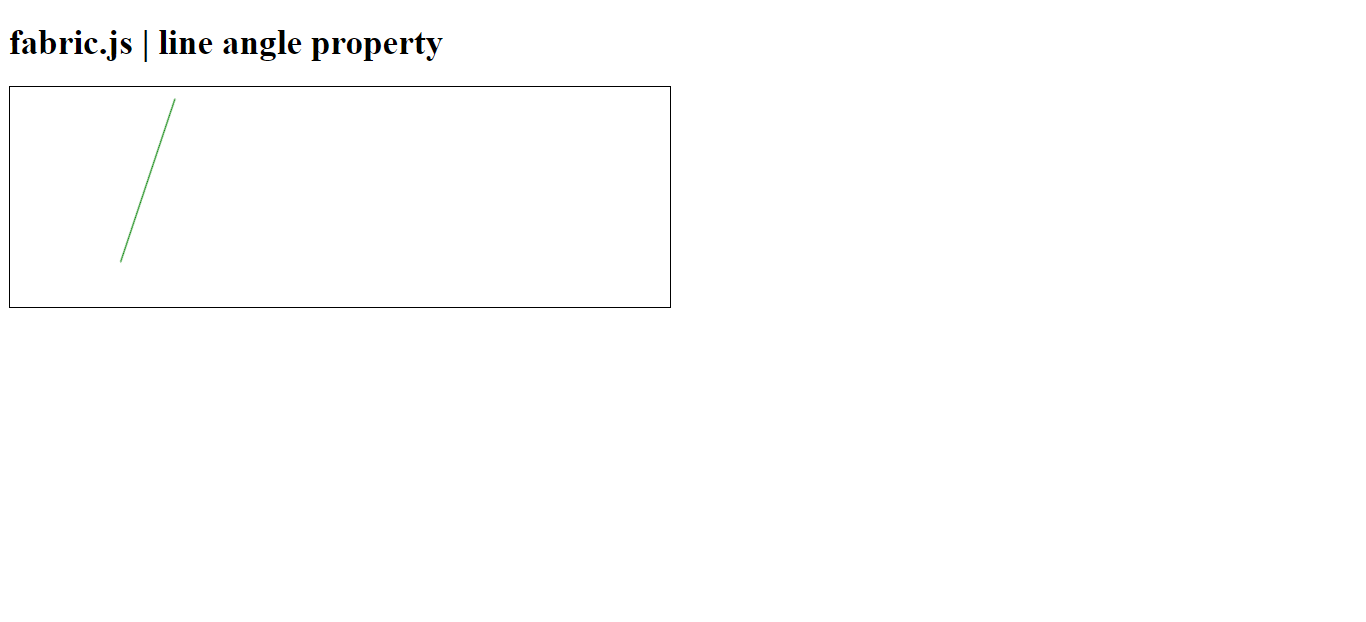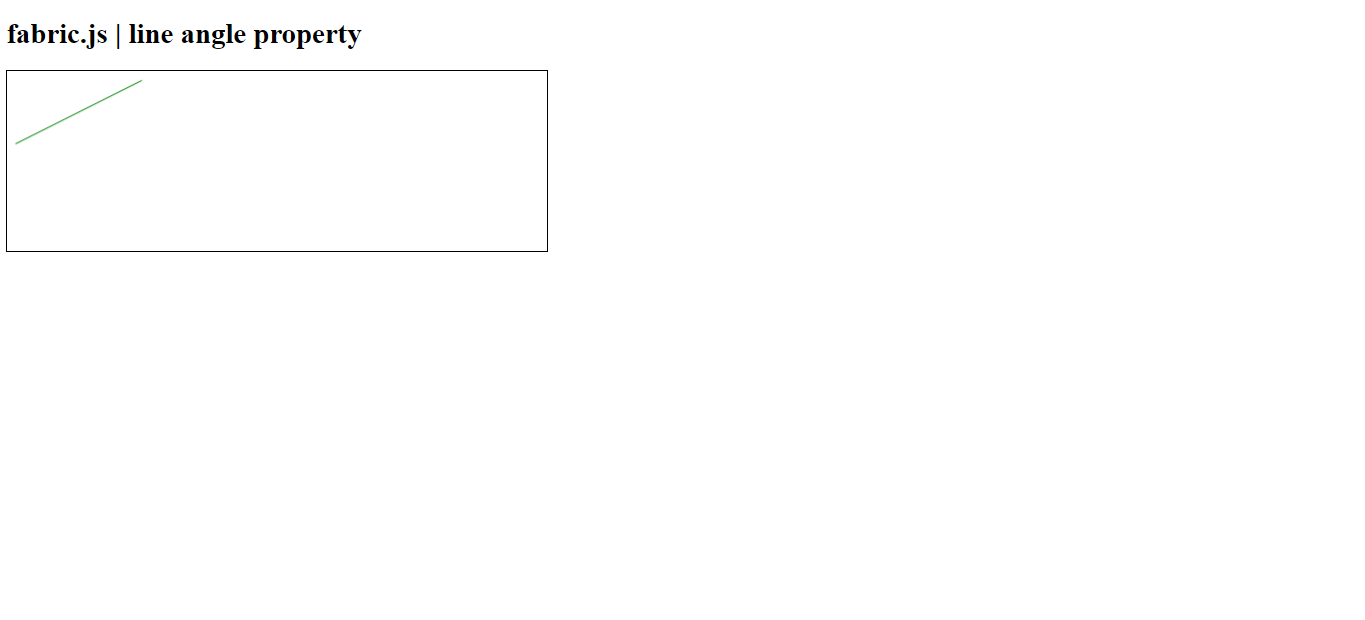Related Articles
Fabric.js line angle Property
• Last Updated : 20 Jan, 2021

In this article, we are going to see how to set the angle of the canvas line using FabricJS. The canvas line means the line is movable and can be stretched according to requirements. Further, the line can be customized when it comes to initial stroke color, height, width, fill color, or stroke width.

To make it possible, we are going to use a JavaScript library called FabricJS. After importing the library, we will create a canvas block in the body tag which will contain the line. After this, we will initialize instances of Canvas and line provided by FabricJS and change the background color of the canvas line using backgroundColor property and render on the Canvas as given in the example below.

Syntax:

```fabric.line({
angle: number
});```

Parameters: This property accepts a single parameter as mentioned above and described below:

• angle: This parameter defines the angle of the line.

Example 1: This example uses FabricJS to enable the angle of the canvas-like line as given below. Try angle the object after enabling angle property, it will rotate the triangle by a defined number. The angle is set to 45 degrees.

## HTML

 ` ``<``html``> `` ` `<``head``> ``   ``<``script` `src``= ``"https://cdnjs.cloudflare.com/ajax/libs/fabric.js/3.6.2/fabric.min.js"``> ``   `` `` `` ` `<``body``> ``   ``<``h1``>fabric.js | line angle property``   ``<``canvas` `id``=``"canvas"` `width``=``"600"` `height``=``"200"``      ``style``=``"border:1px solid #000000;"``> ``   `` ``    ` `   ``<``script``> ``      ``var canvas = new fabric.Canvas("canvas"); ``      ``var line = new fabric.Line([150, 10, 220, 150], { ``         ``stroke: 'green',``         ``angle: 45``      ``}); ``      ``canvas.add(line); ``   `` `` `` ` ``

Output:Example 2: In this example, the angle is set to 90 degrees.

## HTML

 ` ``<``html``> `` ` `<``head``> ``   ``<``script` `src``= ``"https://cdnjs.cloudflare.com/ajax/libs/fabric.js/3.6.2/fabric.min.js"``> ``   `` `` `` ` `<``body``> ``   ``<``h1``>fabric.js | line angle property``   ``<``canvas` `id``=``"canvas"` `width``=``"600"` `height``=``"200"``      ``style``=``"border:1px solid #000000;"``> ``   `` `` ` `   ``<``script``> ``      ``var canvas = new fabric.Canvas("canvas"); ``      ``var line = new fabric.Line([150, 10, 220, 150], { ``         ``stroke: 'green',``         ``angle: 90``      ``}); ``      ``canvas.add(line); ``   `` `` `` ` ``

Output:My Personal Notes arrow_drop_up